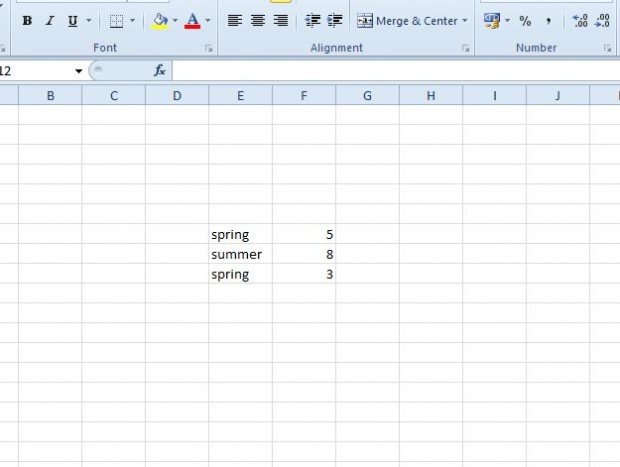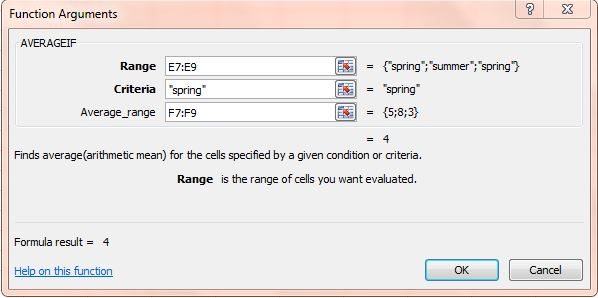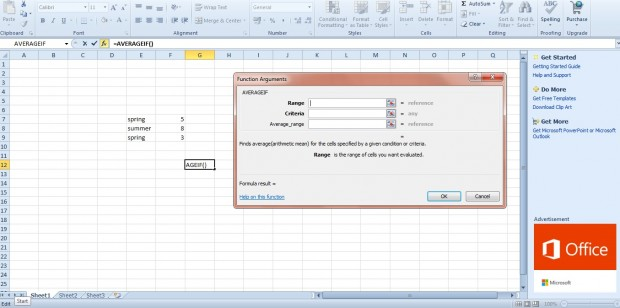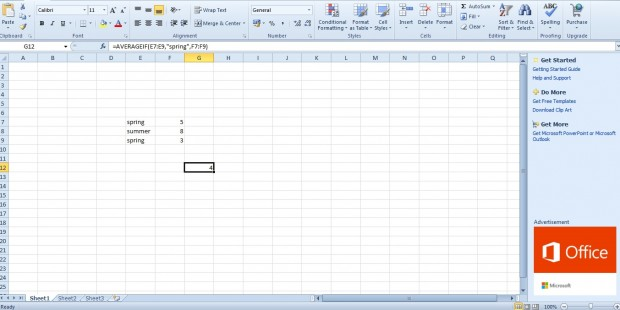# How to use AVERAGEIF function in Excel spreadsheets [Tip]

AVERAGEIF is an Excel 2010/13 function that returns average values based on specified criteria. For example, if you have a data table you can add an AVERAGEIF function to a cell to find average values from one column that match criteria in another. The function’s syntax is: AVERAGEIF( range, criteria, [average_range] ).

So let’s try this function out with an example. Enter spring, summer, spring and 5, 8, 3 in two adjacent columns. Then the Excel spreadsheet should match the one below.Now select a cell to add the AVERAGEIF function to. Press fx to open the Insert Function window, and select the AVERAGEIF function. That opens the AVERAGEIF window shown below.Press the button beside the Range field, and select the cells with spring, summer and spring in them. Enter spring in the Criteria text box. Then press the button beside the Average_range text box to select the column with the numerical values in it.Press OK to add the function to the selected cell. It should return a value of 4 as in the snapshot below. Thus, the function has found the average value of all those cells that are in the same row as the spring criteria. Five + three amounts to eight, which when divided by two gives you an average value of four for those two cells. If you switched the criteria to summer, the AVERAGEIF cell would return a value of 8.This can be a handy function to add to spreadsheets that include data tables. Note that the function was introduced in Excel 2007, so is not available in every Excel version. You can play this YouTube video for further details on how to add the AVERAGEIF function to spreadsheets.